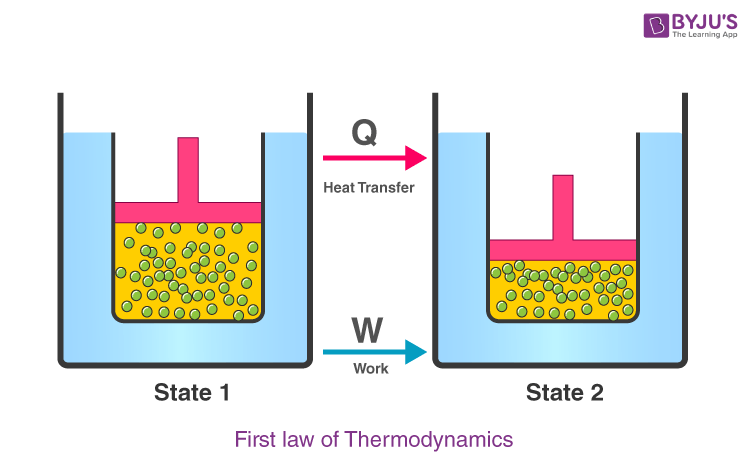# First Law Of Thermodynamics

The First Law of Thermodynamics states that heat is a form of energy, and thermodynamic processes are therefore subject to the principle of conservation of energy. This means that heat energy cannot be created or destroyed. It can, however, be transferred from one location to another and converted to and from other forms of energy.

## Introducing State Variables

Thermodynamic state variables are the macroscopic quantities which determine the thermodynamic equilibrium state of a system. A system not in equilibrium cannot be described by state variables. State variables can further be classified as intensive or extensive variables. Intensive variables are independent of the dimensions of the system like pressure and temperature, while extensive variables depend on dimensions of the system like volume, mass, internal energy etc.

### Explaining the first law of thermodynamics

The first law of thermodynamics relates to heat, internal energy, and work.

The first law of thermodynamics, also known as the law of conservation of energy states that energy can neither be created nor destroyed, but it can be changed from one form to another.According to this law, some heat given to the system is used to change the internal energy while the rest is used in doing work by the system.

It can be represented mathematically as

 $\Delta Q=\Delta U+W$

Where,

• ΔQ is the heat given or lost
• ΔU is the change in internal energy
• W is the work done

We can also represent the above equation as follows,

 $\Delta U=\Delta Q-W$

So we can infer from the above equation that the quantity (ΔQ – W) is independent of the path taken to change the state. Further, we can say that internal energy tends to increase when the heat is given to the system and vice – versa.

### Sign Conventions

The table below shows the appropriate sign conventions for all three quantities under different conditions:

 ΔU (change in internal energy) Q (heat) W (work done on the gas) is “+” if temperature increases is “+” if heat enters gas is “+” if gas is compressed is “-” if temperature decreases is “-” if heat exist gas is “-” if gas expands is “0” if temperature is constant is “0” if no heat is exchanged is “0” if volume is constant

## First Law of Thermodynamics Solved Examples

1. Calculate the change in the internal energy of the system if 3000 J of heat is added to a system and a work of 2500 J is done.

Solution:
The following sign conventions are followed in the numerical:
Solution:
The following sign conventions are followed in the numerical:

• Q is positive as heat is added to the system
• W is positive if work is done on the system

Hence, the change in internal energy is given as:

$\Delta U=3000-2500$

$\Delta U=500$

The internal energy of the system is 500 J.

2. What is the change in the internal energy of the system if 2000 J of heat leaves the system and 2500 J of work is done on the system?
Solution:
The change in the internal energy of the system can be identified using the formula:

ΔU = Q-W

Substituting the values in the following equation, we get

ΔU = -2000-(-3000)

ΔU = -2000+3000

ΔU = 1000 Joule

Internal energy increases by 4500 Joules.

## Frequently Asked Questions – FAQs

### What does the first law of thermodynamics state?

The first law of thermodynamics states that energy can neither be created nor destroyed, it merely transforms from one form to another.

### Who stated the first law of thermodynamics?

Rudolf Clausius and William Thomson stated the first law of thermodynamics.

### Can the first law of thermodynamics be violated?

A machine called a Perpetual Motion Machine of the first kind violates the first law by creating energy.

### Why is the first law of thermodynamics important to the environment?

The first law states that energy can neither be created nor destroyed it can only e transformed from one form to another. Sun is the only source of energy for all living organisms on Earth. This solar energy is converted into chemical energy by plants through the process of photosynthesis. These energies obtained by the plants do not go back into the solar system, rather it is passed on to herbivores that feed on green plants. Some part of the energy obtained by the herbivores is utilized by carnivores or transferred to the decomposers when the herbivores die.

### What are the limitations of the first law of thermodynamics

The first law of thermodynamics does not quantify the energy transfer that takes place failing to explain the feasibility of the thermal process.

Stay tuned with BYJU’S for more such interesting articles. Also, register to “BYJU’S – The Learning App” for loads of interactive, engaging Physics-related videos and an unlimited academic assist.

#### 1 Comment

1. saurabh singh

it is important notes